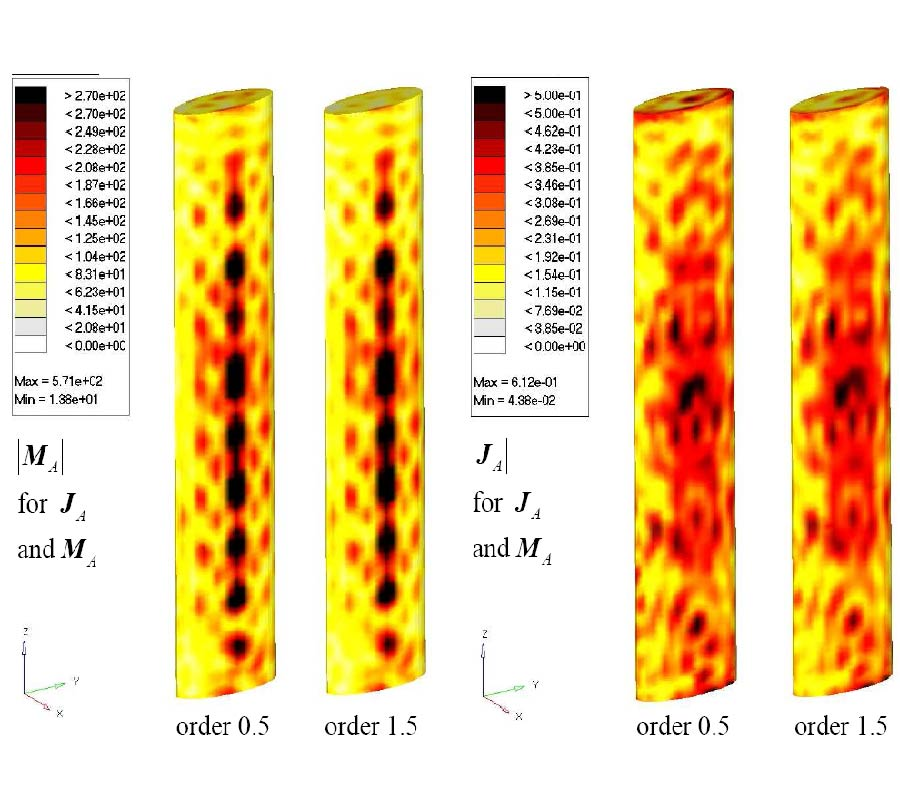# Vol. 106

Latest Volume
All Volumes
All Issues
2010-07-28

#### Inverse Equivalent Surface Current Method with Hierarchical Higher Order Basis Functions, Full Probe Correction and Multilevel Fast Multipole Acceleration (Invited Paper)

By Thomas F. Eibert, Ismatullah, E. Kaliyaperumal, and Carsten H. Schmidt
Progress In Electromagnetics Research, Vol. 106, 377-394, 2010
doi:10.2528/PIER10061604

## Abstract

An inverse equivalent surface current method working with equivalent electric and/or magnetic surface current densities on appropriately chosen Huygens surfaces is investigated. The considered model with triangular surface meshes is compatible with the models known from method of moments (MoM) solutions of surface integral equations. Divergence conforming current basis functions of order 0.5 and of order 1.5 are considered, where the order 0.5 functions are the well-known Rao-Wilton-Glisson basis functions. Known near-field samples typically obtained from measurements are mapped on the unknown equivalent surface current densities utilizing the radiation integrals of the currents as forward operator, where the measurement probe influence is formulated in a MoM like weighting integral. The evaluation of the forward operator is accelerated by adaptation of the multilevel fast multipole method (MLFMM) to the inverse formulation, where the MLFMM representation is the key to full probe correction by employing only the far-field patterns of the measurement probe antennas. The resulting fully probe corrected algorithm is very flexible and efficient, where it is found that the computation speed is mostly dependent on the MLFMM configuration of the problem and not that much on the particular equivalent current expansion as long as the expansion is able to represent the currents sufficiently well. Inverse current and far-field pattern results are shown for a variety of problems, where near-field samples obtained from simulations as well as from realistic measurements are considered.

## Citation

Thomas F. Eibert, Ismatullah, E. Kaliyaperumal, and Carsten H. Schmidt, "Inverse Equivalent Surface Current Method with Hierarchical Higher Order Basis Functions, Full Probe Correction and Multilevel Fast Multipole Acceleration (Invited Paper)," Progress In Electromagnetics Research, Vol. 106, 377-394, 2010.
doi:10.2528/PIER10061604
http://test.jpier.org/PIER/pier.php?paper=10061604

## References

1. Yaghjian, A., "An overview of near-field antenna measurements," IEEE Trans. Antennas Propag., Vol. 34, No. 1, 30-45, 1986.

2. Hansen, J. E., Spherical Near-field Antenna Measurements, IEE Electromagnetic Wave Series 26, 1988.

3. Kerns, D. M., "Plane-wave scattering-matrix theory of antennas and antenna-antenna interactions," National Bureau of Standards, Boulder CO, 1981.

4. Cappellin, C., O. Breinbjerg, and A. Frandsen, "Properties of the transformation from the spherical wave expansion to the plane wave expansion," Radio Sci., Vol. 43, No. 1, 2008.

5. Sarkar, T. and A. Taaghol, "Near-field to near/far-field transformation for arbitrary near-field geometry utilizing an equivalent electric current and MoM," IEEE Trans. Antennas Propag., Vol. 47, No. 3, 566-573, 1999.

6. Alvarez, Y., F. Las-Heras, and M. R. Pino, "Reconstruction of equivalent currents distribution over arbitrary three-dimensional surfaces based on integral equation algorithms," IEEE Trans. Antennas Propag., Vol. 55, No. 12, 3460-3468, 2007.

7. Eibert, T. F. and C. H. Schmidt, "Multilevel fast multipole accelerated inverse equivalent current method employing Rao-Wilton-Glisson discretization of electric and magnetic surface currents," IEEE Trans. Antennas Propag., Vol. 57, No. 4, 1178-1185, 2009.

8. Rao, S. M., D. R. Wilton, and A. W. Glisson, "Electromagnetic scattering by surfaces of arbitrary shape," IEEE Trans. Antennas Propag., Vol. 30, No. 3, 409-418, 1982.

9. Petre, P. and T. K. Sarkar, "Planar near-field to far-field transformation using an equivalent magnetic current approach," IEEE Trans. Antennas Propag., Vol. 40, No. 11, 1348-1356, 1992.

10. Alvarez, Y., F. Las-Heras, M. R. Pino, and J. A. Lopez, "Acceleration of the sources reconstruction method via the fast multipole method," IEEE Antennas Propag. Intern. Symp., 2008.

11. Lopez, Y. A., F. Las-Heras, and M. R. Pino, "Application of the adaptive cross approximation algorithm to the sources reconstruction method," European Conf. Antennas Propag., 2009.

12. Eibert, T. F., "A diagonalized multilevel fast multipole method with spherical harmonics expansion of the k-space integrals," IEEE Trans. Antennas Propag., Vol. 53, No. 2, 814-817, 2005.

13. Tzoulis, A. and T. F. Eibert, "Efficient electromagnetic near-field computation by the multilevel fast multipole method employing mixed near-field/far-field translations," IEEE Antennas Wireless Propag. Lett., Vol. 4, 449-452, 2005.

14. Chew, W. C., T. J. Cui, and J. M. Song, "A FAFFA-MLFMA algorithm for electromagnetic scattering," EEE Trans. Antennas Propag., Vol. 50, No. 11, 1641-1649, 2002.

15. Jorgensen, E., J. L. Volakis, P. Meincke, and O. Breinbjerg, "Higher order hierarchical Legendre basis functions for electromagnetic modeling," IEEE Trans. Antennas Propag., Vol. 52, No. 11, 2985-2995, 2004.

16. Sun, D. K., J. F. Lee, and Z. Cendes, "Construction of nearly orthogonal Nedelec bases for rapid convergence with multilevel preconditioned solvers," SIAM J. Sci. Comput., Vol. 23, No. 4, 1053-1076, 2001.

17. Ismatullah and T. F. Eibert, "Surface integral equation solutions by hierarchical vector basis functions and spherical harmonics based multilevel fast multipole method," IEEE Trans. Antennas Propag., Vol. 57, No. 7, 2084-2093, 2009.

18. Schmidt, C. H., M. M. Leibfritz, and T. F. Eibert, "Fully probe-corrected near-field far-field transformation employing plane wave expansion and diagonal translation operators," IEEE Trans. Antennas Propag., Vol. 56, No. 3, 737-746, 2008.

19. Araque Quijano, J. L. and G. Vecchi, "Field and source equivalence in source reconstruction on 3D surfaces," Progress In Electromagnetics Reseach, Vol. 103, 67-100, 2010.

20. Chew, W. C., J.-M. Jin, and E. Michielssen, Fast and Effcient Algorithms in Computational Electromagnetics, Artech House, Boston, 2001.

21. Saad, Y., Iterative Methods for Sparse Linear Systems, PWS, Boston, 1996.

22. Bjorck, A., Numerical Methods for Least Squares Problems, SIAM, Philadelphia, 1996.

23. Las-Heras, F., B. Galocha, and J. L. Besada, "Equivalent source modelling and reconstruction for antenna measurement and synthesis," IEEE Antennas Propag. Intern. Symp., 2007.